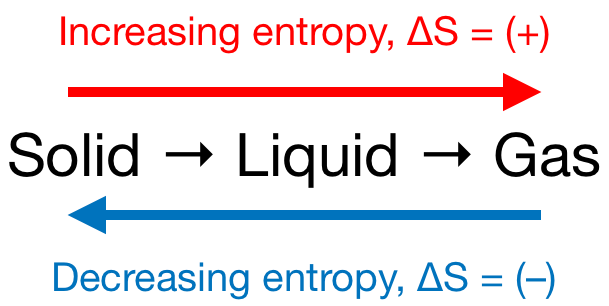# Problem: Consider the following reaction 2 SO2(g) + O2(g) → 2 SO3(g)The free energies of formation at 25°C are given below:SO2 = -300 kJ/molSO3 = -371 kJ/mola) Is entropy change for the reaction negative, positive, or 0? Explain.b) Is the enthalpy change for the reaction negative, positive, or zero? Explain.c) Is the reaction spontaneous at 25°C. Explain why or why not.d) Is the reaction spontaneous at all temperatures? Explain your answer.e) Is the value of the equilibrium constant for the reaction at 298 K less than one, zero, or greater than one. Show your reasoning.

###### FREE Expert Solution

We're given the following reaction:

2 SO2(g) + O2(g) → 2 SO3(g)

For Part a):

Recall that if:

• ΔS > 0 or ΔS = (+); entropy is increased
• ΔS < 0 or ΔS = (–); entropy is decreased

Remember that phase changes lead to a change in entropy:99% (268 ratings)###### Problem Details

Consider the following reaction 2 SO2(g) + O2(g) → 2 SO3(g)

The free energies of formation at 25°C are given below:

SO2 = -300 kJ/mol
SO3 = -371 kJ/mol

a) Is entropy change for the reaction negative, positive, or 0? Explain.

b) Is the enthalpy change for the reaction negative, positive, or zero? Explain.

c) Is the reaction spontaneous at 25°C. Explain why or why not.